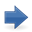### Best Techniques Leave Little Room for Substitution. A New Critique of the Production Function

CSWP 47 (December 2020)

Author Götz Kersting and Bertram Schefold

Keywords Capital theory; production function; substitution; reswitching.

JEL B24; C62; C67; D57.

Samuelson assumed a linear wage curve for each of a continuum of techniques such that their envelope was a monotonically falling wage curve for the economy, from which an aggregate production function fulfilling the marginal productivity conditions could be derived. But the capital intensities of the techniques chosen at each rate of profit are not necessarily lower at higher rates of profit, if the wage curves are not linear, a possibility exemplified by reswitching. This critique of the capital controversy does not rule out Samuelson’s construction as an approximation, since the paradoxes have been shown to be rare. Instead, a possibility is likely that has so far not been noticed: the envelope of the wage curves will in the relevant range of the rate of profit be dominated by a small number of efficient techniques of approximately equal capital intensity, leaving little room for substitution. Anew mathematical theorem demonstrates that the expected number of techniques that appear on the envelope is given by (2/3) ln s.Download the paper